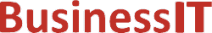O nás     Inzerce     KontaktSpolehlivé informace o IT již od roku 2011[Linux manuál]

# fmod, fmodf, fmodl: Funkce s plovoucí desetinnou čárkou

Originální popis anglicky: fmod, fmodf, fmodl - floating-point remainder value function

Návod, kniha: POSIX Programmer's Manual

## STRUČNĚ

#include <math.h>

double fmod(double x, double y);

float fmodf(float x, float y);

long double fmodl(long double x, long double y);

## POPIS / INSTRUKCE

These functions shall return the floating-point remainder of the division of x by y.
An application wishing to check for error situations should set errno to zero and call feclearexcept(FE_ALL_EXCEPT) before calling these functions. On return, if errno is non-zero or fetestexcept(FE_INVALID | FE_DIVBYZERO | FE_OVERFLOW | FE_UNDERFLOW) is non-zero, an error has occurred.

## NÁVRATOVÁ HODNOTA

These functions shall return the value x- i* y, for some integer i such that, if y is non-zero, the result has the same sign as x and magnitude less than the magnitude of y.
If the correct value would cause underflow, and is not representable, a range error may occur, and  either 0.0 (if supported), or  an implementation-defined value shall be returned.
If x or y is NaN, a NaN shall be returned.
If y is zero, a domain error shall occur, and either a NaN (if supported), or an implementation-defined value shall be returned.
If x is infinite, a domain error shall occur, and either a NaN (if supported), or an implementation-defined value shall be returned.
If x is ±0 and y is not zero, ±0 shall be returned.
If x is not infinite and y is ±Inf, x shall be returned.
If the correct value would cause underflow, and is representable, a range error may occur and the correct value shall be returned.

## CHYBY / ERRORY

These functions shall fail if:
Domain Error
The x argument is infinite or y is zero.
If the integer expression (math_errhandling & MATH_ERRNO) is non-zero, then errno shall be set to [EDOM]. If the integer expression (math_errhandling & MATH_ERREXCEPT) is non-zero, then the invalid floating-point exception shall be raised.

These functions may fail if:
Range Error
The result underflows.
If the integer expression (math_errhandling & MATH_ERRNO) is non-zero, then errno shall be set to [ERANGE]. If the integer expression (math_errhandling & MATH_ERREXCEPT) is non-zero, then the underflow floating-point exception shall be raised.

The following sections are informative.

None.

## APPLICATION USAGE

On error, the expressions (math_errhandling & MATH_ERRNO) and (math_errhandling & MATH_ERREXCEPT) are independent of each other, but at least one of them must be non-zero.

None.

None.

## SOUVISEJÍCÍ

feclearexcept() , fetestexcept() , isnan() , the Base Definitions volume of IEEE Std 1003.1-2001, Section 4.18, Treatment of Error Conditions for Mathematical Functions, <math.h> Portions of this text are reprinted and reproduced in electronic form from IEEE Std 1003.1, 2003 Edition, Standard for Information Technology -- Portable Operating System Interface (POSIX), The Open Group Base Specifications Issue 6, Copyright (C) 2001-2003 by the Institute of Electrical and Electronics Engineers, Inc and The Open Group. In the event of any discrepancy between this version and the original IEEE and The Open Group Standard, the original IEEE and The Open Group Standard is the referee document. The original Standard can be obtained online at http://www.opengroup.org/unix/online.html .
 2003 IEEE/The Open Group
©2011-2020 BusinessIT.cz, ISSN 1805-0522 | Názvy použité v textech mohou být ochrannými známkami příslušných vlastníků.
Provozovatel: Bispiral, s.r.o., kontakt: BusinessIT(at)Bispiral.com | Inzerce: Best Online Media, s.r.o., zuzana@online-media.cz
O vydavateli | Pravidla webu BusinessIT.cz a ochrana soukromí | pg(8908)